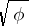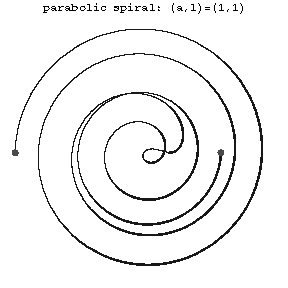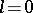# Parabolic spiral

(diff) ← Older revision | Latest revision (diff) | Newer revision → (diff)

A transcendental plane curve whose equation in polar coordinates has the formTo each value ofcorrespond two values of, one positive and one negative.Figure: p071250a

The curve has infinitely many double points and one point of inflection (see Fig.). If, then the curve is called the Fermat spiral. The parabolic spiral is related to the so-called algebraic spirals (see Spirals).

How to Cite This Entry:
Parabolic spiral. Encyclopedia of Mathematics. URL: http://encyclopediaofmath.org/index.php?title=Parabolic_spiral&oldid=19135
This article was adapted from an original article by D.D. Sokolov (originator), which appeared in Encyclopedia of Mathematics - ISBN 1402006098. See original article